### A more involved example using frequency filters

This second example has as an aim to illustrate the differences between simple and more complex filters.  In the first example we didn't need any particular performance of the filter to separate the small signal band from the broad noise band, and in any case, there remained noise in the signal band.   This time we study the case where a useful signal in a relatively narrow band is squeezed between two narrow noise bands, but without any overlap.  This is the typical case with radio, where a radio signal in a specific band has to be separated from nearby other channels.  The performance of the band pass filter will hence be primordial in the result.

In the example we use relatively simple mathematical formulas for the signal and the "noise", although of course this cannot be the case in a true problem: if we would know the formula of the signal, then we wouldn't need to receive it !

The signal is s(t), and the noise consists of two components: s2a(t) and s2b(t).  t is in micro seconds.

```s = cos(180.02*t)*sin(8.81*sin(t)+2*t);
s2a = cos(230.3*t)*sin(7.9*sin(t)+5*t);
s2b = cos(130.3*t)*sin(7.9*sin(t)+5*t);
s2 = s2a + s2b;```

The signal and the noise look like this: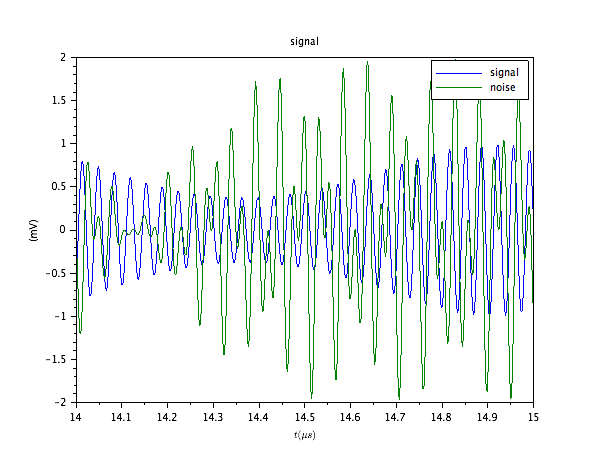The noisy signal is then: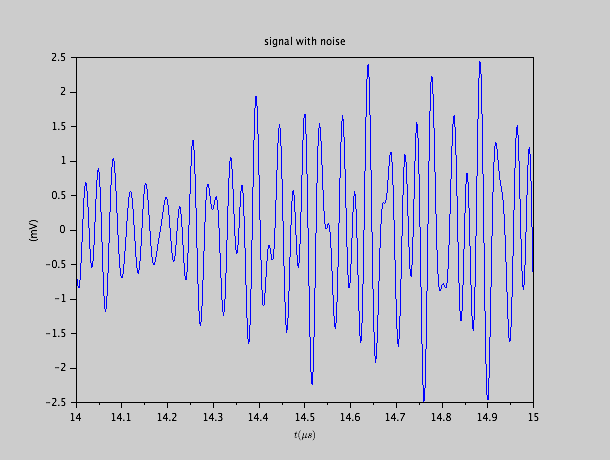We hardly recognize the beat of the original signal.

The spectral densities show us that the signal resides in a band that is essentially clear of any perturbation, but with nearby noise bands.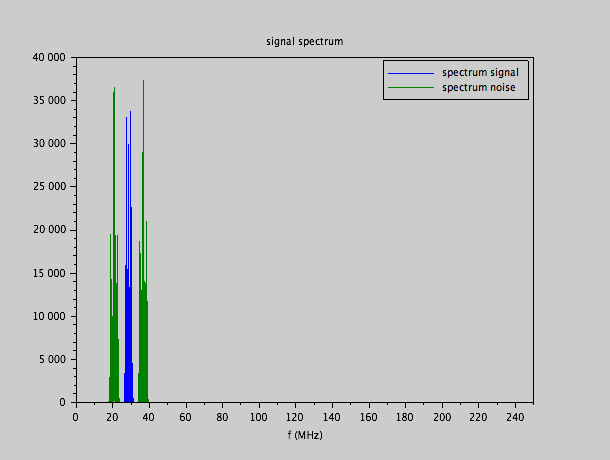This implies that a filter that would perfectly cut out the band from 25 MHz to 32 Mhz would allow us to reconstruct the original signal perfectly.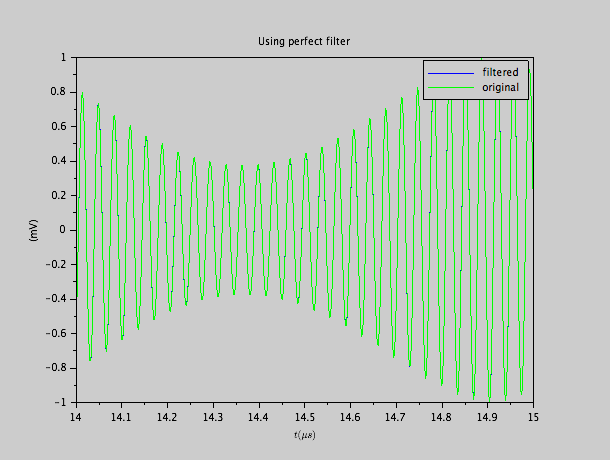We observe indeed that the original signal, and the noisy signal passed through the perfect band filter, are identical.  As in the first example, we could use a perfect filter, because we had recorded the entire signal.  A Fourier transform of the entire signal allows us to keep exactly that portion of the spectrum we are interested in, and an inverse Fourier transform then reconstructs the signal as if it passed through a prefect band pass filter.

We will now construct a third order Butterworth, Chebyshev I, and Elliptic filter with a pass band from 25 MHz to 32 MHz, and apply it to the noisy signal.  However, in order to observe the performance of the filters, we applied a shift in time so that the filtered result coincides best with the original looked-for signal.  In other words, we corrected, in the plot, for the delay caused by the realistic filters.  That delay is different from filter to filter.  It turns out to be 106 ns for the Butterworth filter, and 72 ns for the Elliptic and Chebyshev I filter.

The result indicates us that indeed, most of the signal is recovered, but the separation is not total: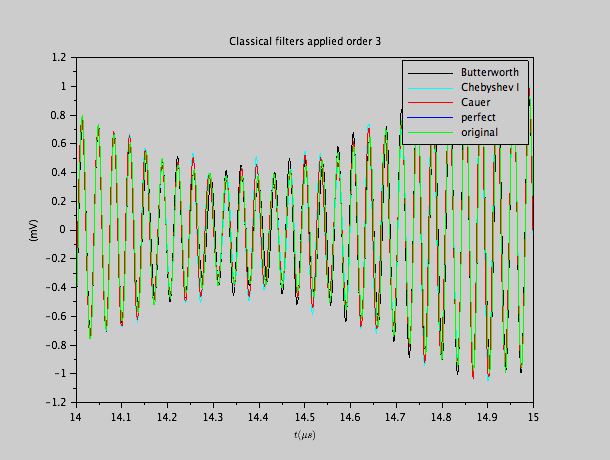Depending on the application, this can be good enough, or not.

If we increase the order to 6, we obtain a much better result: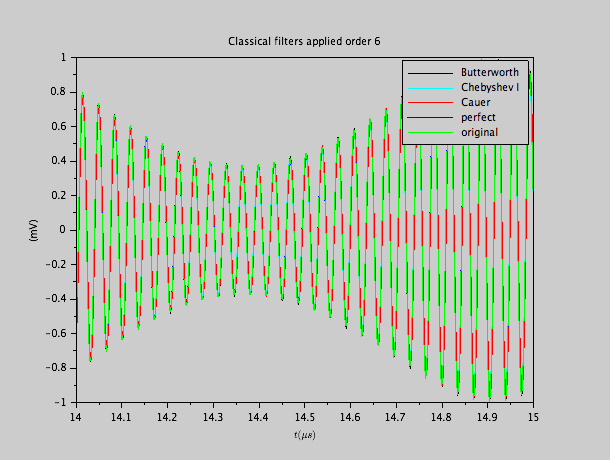The delays have been adapted: they are now 176 ns for the Butterworth filter, 212 ns for the Chebyshev I filter and 140 ns for the elliptic filter.

### Analogue passive filters

If we can accept the results with a third order Butterworth filter, one can build a rather simple passive filter.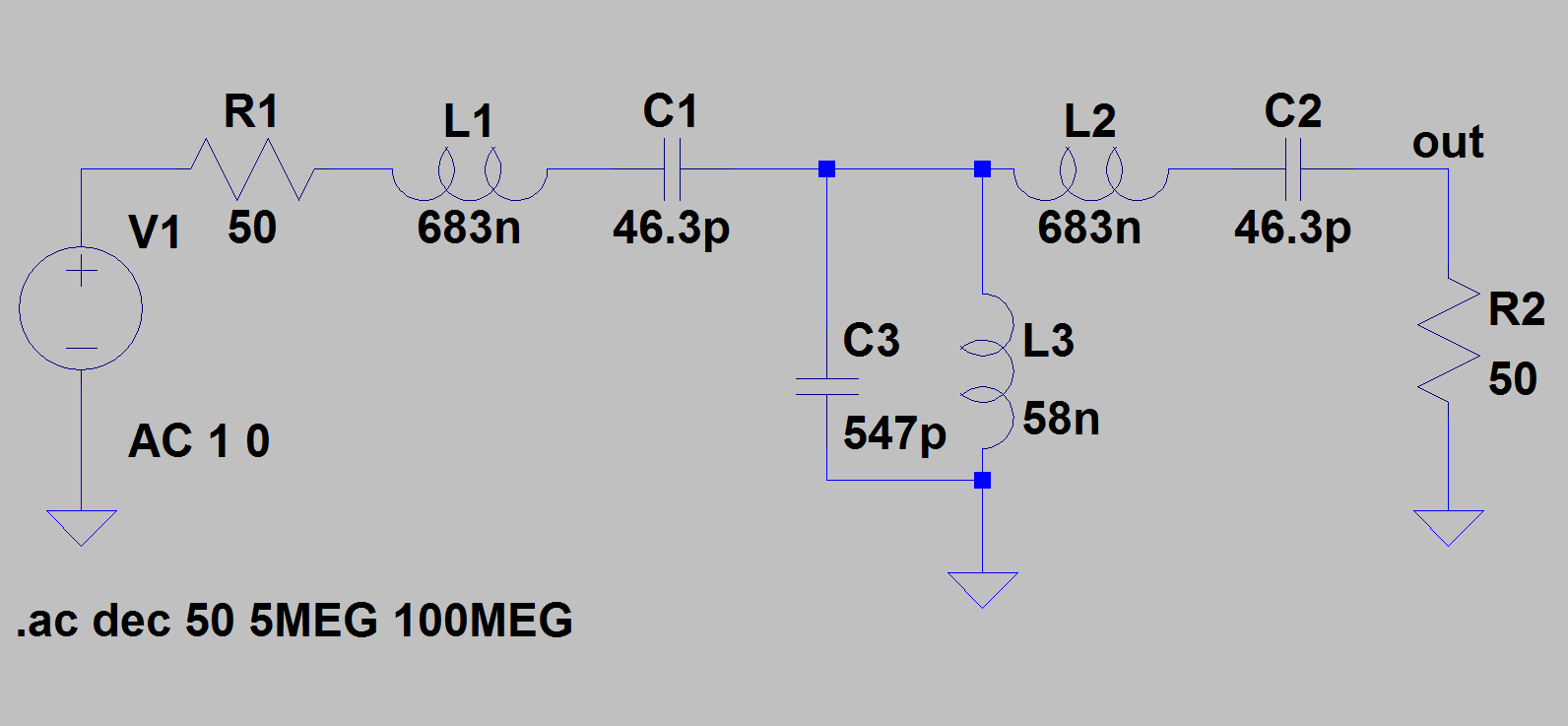As we see, the values of the inductors and the capacitors are very reasonable (these are theoretical values which have to be approximated by commercial values when one wants to build the actual circuit of course).

The response of this filter looks like this: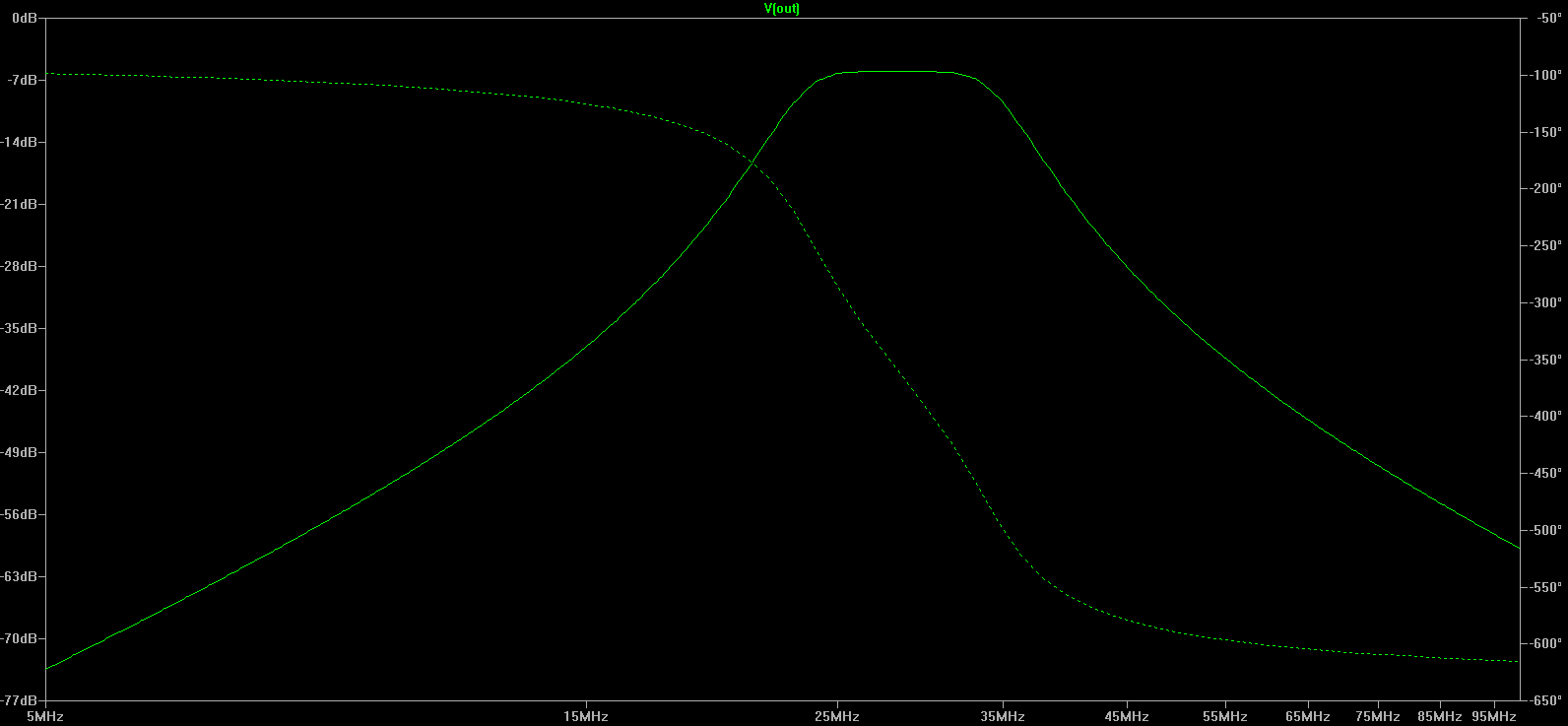Although the pass band is very smooth (this is the fundamental property of a Butterworth filter), the drop off is not very fast.  We can understand that at 20 MHz, still one tenth of the noise signal will pass the filter, which is why the result of this filter was not entirely satisfying.  It depends upon the application whether one can live with this approximation or not.

If one can't, the much better 6th order Elliptic filter is of course much more involved: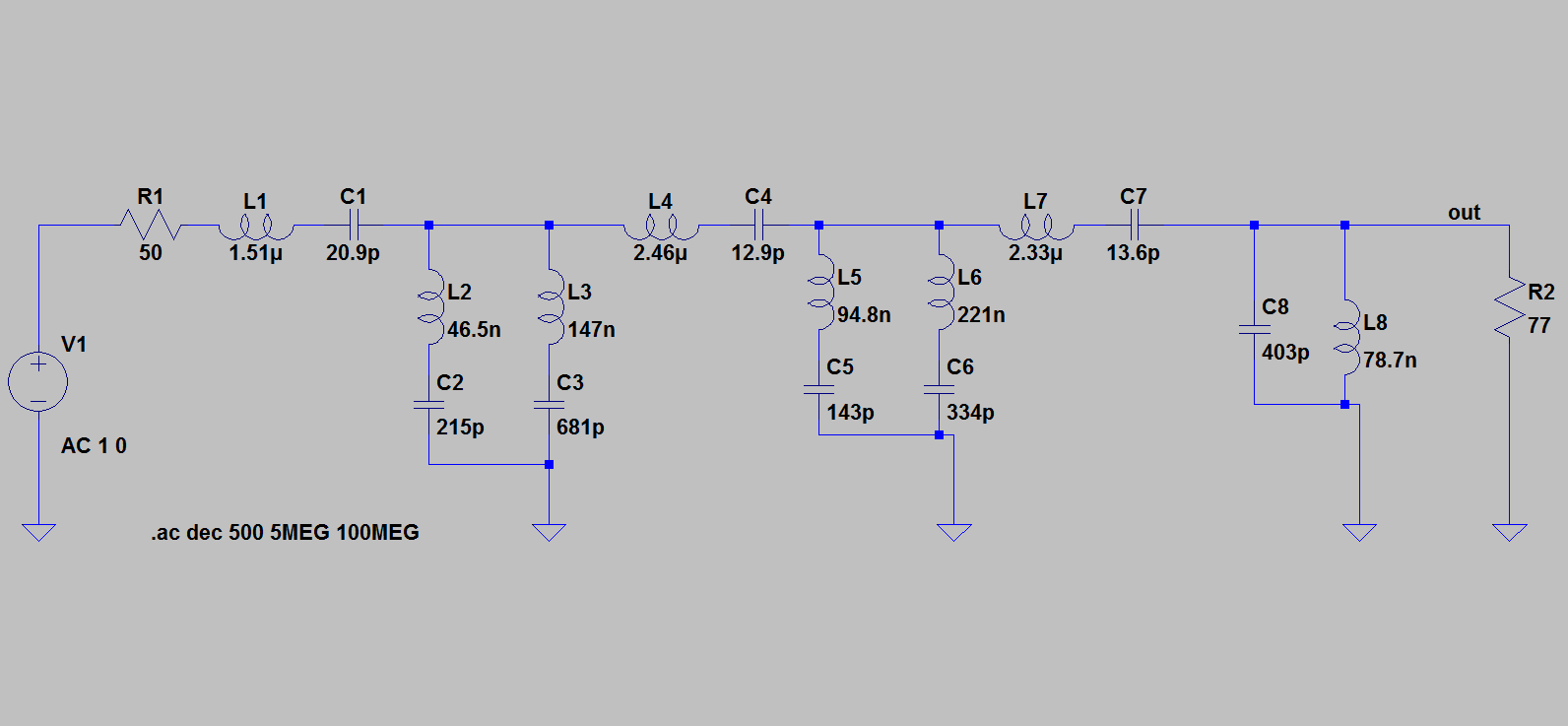The response of the above filter, with ideal capacitors and inductors, looks very impressive: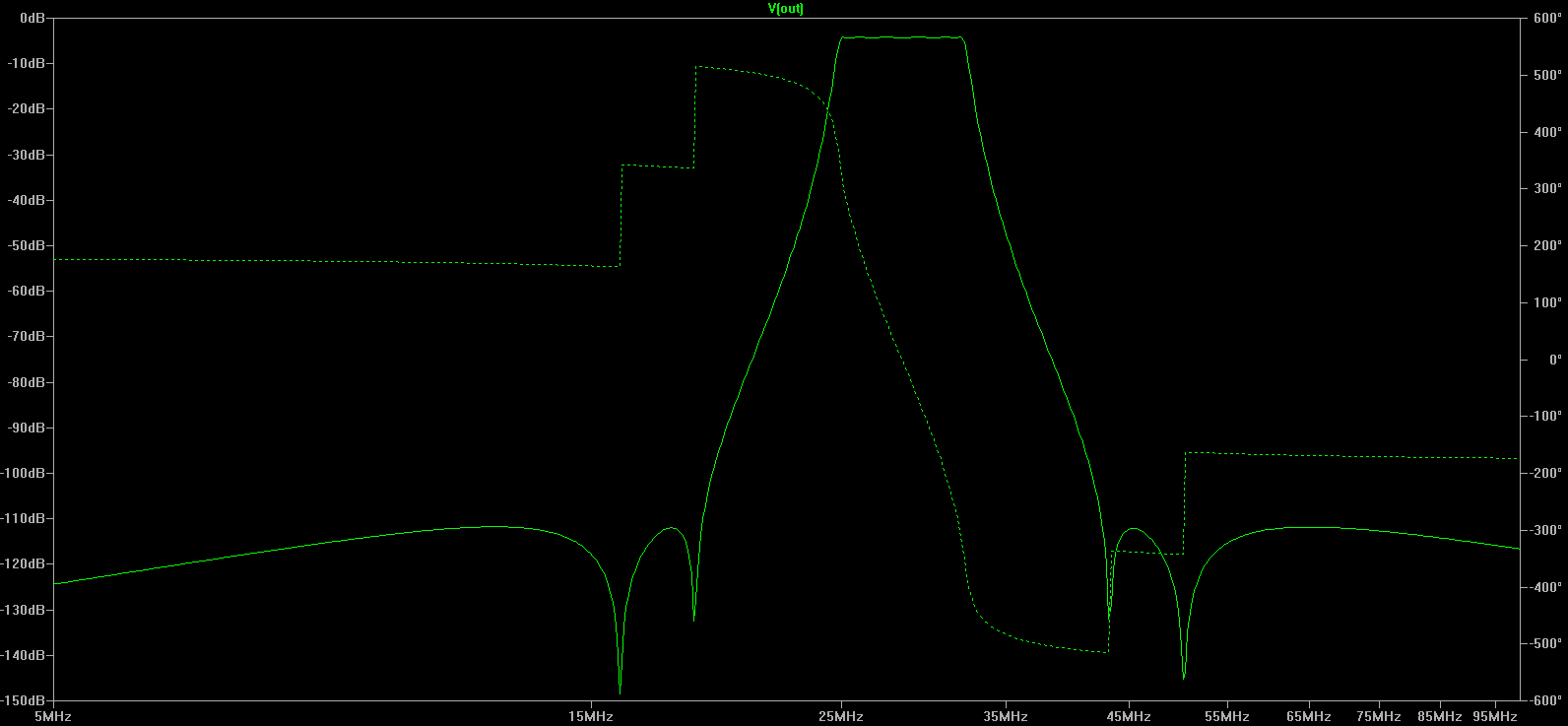We see that the noise band around 20 MHz is suppressed by about 60 dB !

Apart from a study of the non-ideal behavior of the components, this filter is perfectly realizable.  As it is a passive filter made up of mostly non-dissipative elements, it doesn't add any noise, and will be perfectly linear in as much as the inductors behave linearly.  The values of the components are within a very reasonable range.  If one can build such kind of filter, one shouldn't hesitate, in fact.  The question is: can we ?

The problem is the precision of the components required.  If we take the values of the components to be the nearest E12 values (admittedly a somewhat rough approximation), and we assume a series resistance of each component to be 0.1 Ohm, and we take a 1 pF parallel parasitic capacitance on each inductor, our impressive transfer function becomes somewhat less nice: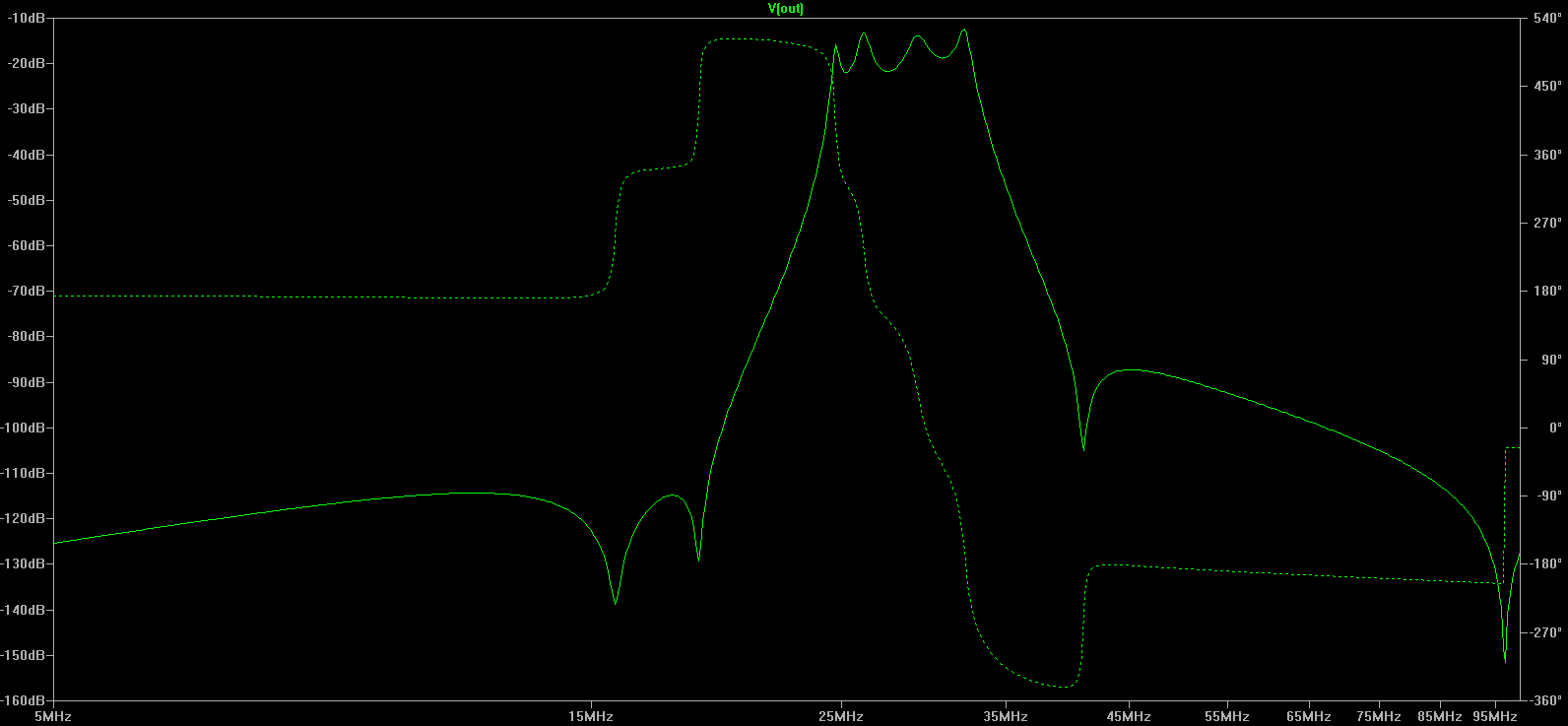In fact, the selectivity is still very good: the rejection of the noise bands is still impressive.  What has deteriorated significantly, however, is the passband.  First of all, there is a bigger loss in the pass band ; but especially, the ripple which was originally specified to be only 0.2 dB, is now more than 10 dB !  This may introduce more significant distortions of the signal, than the deviations due to the noise.   We may in fact prefer the low-order Butterworth filter over this distorted elliptic filter !

It is true that we have been quite sloppy by taking only E12 values, and by considering 0.1 Ohm series resistance of each component: most precision components can do better.  There are less performing components too, however.  This shows us the danger of designing sophisticated passive filters: they require extremely precise and high quality components.  Tolerances on values, rounding to commercial values and parasitic elements can totally ruin the spectacular effect of a high order filter, and it requires significant attention to make such a filter work as expected in reality.

### Active filters

In general, it is easier to find (small) capacitor components, and resistor components which are near-ideal, than inductors.  This is why active filters can suffer less from the above problem of component non-idealities than passive high order filters.   Active filters can be constructed in many different ways, and it is not always the circuit with the simplest topology and the fewest operational amplifiers that is the easiest to build in such a way that the performance is satisfying.

First we make a 6th order Cauer filter with the simplest (lowest op amp count) topologies: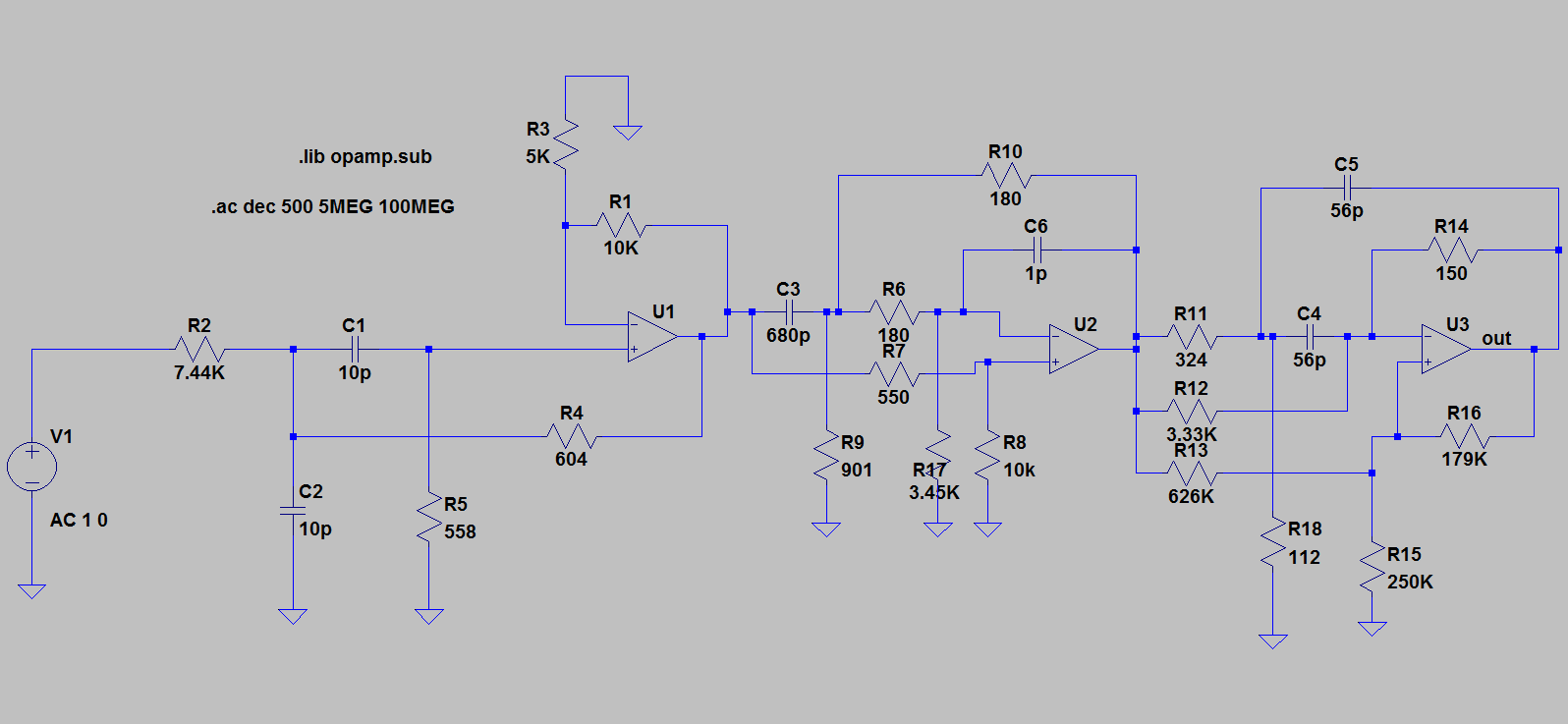Its response is: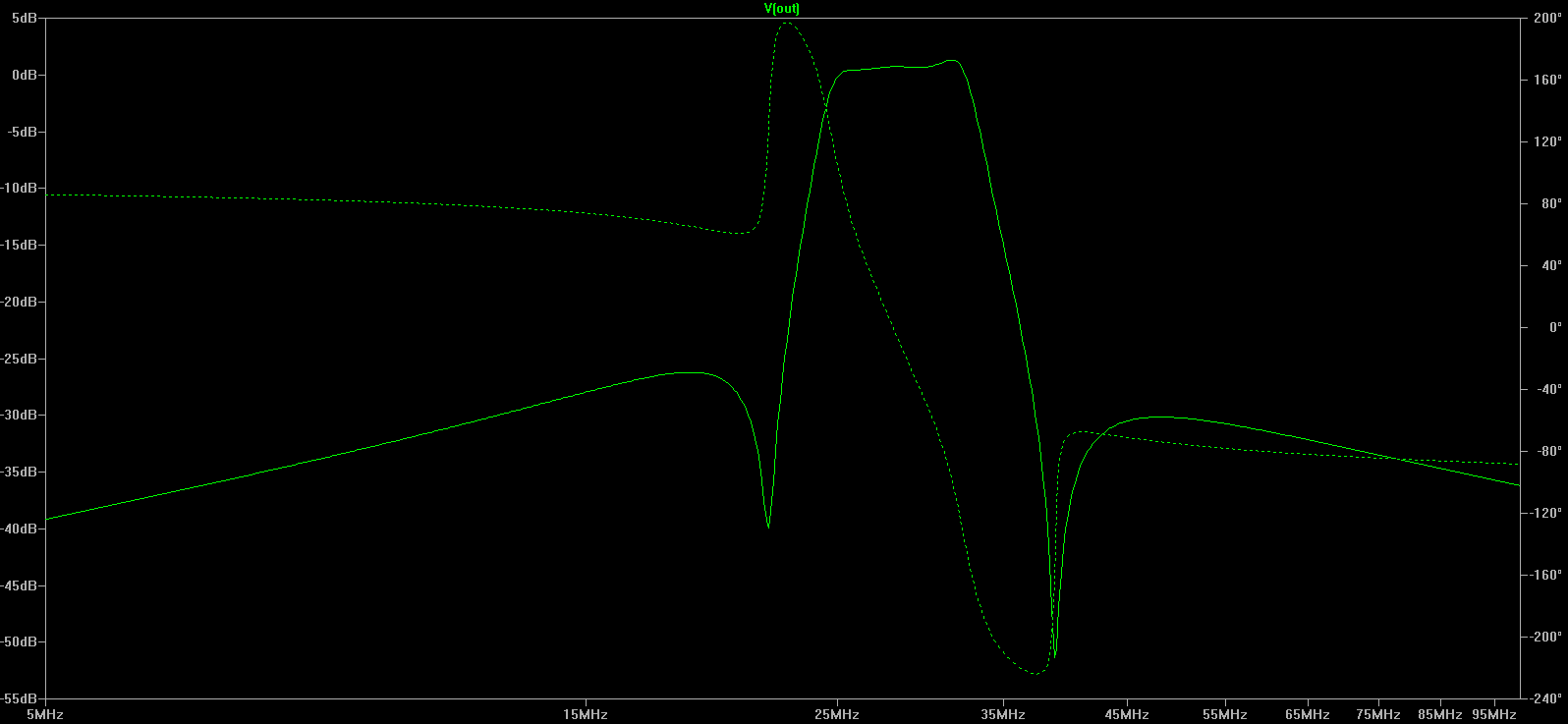The response looks all right, except that the cutoff is not very low ; in fact, this is because we really made a 6th order band pass filter, while the passive filter is in fact a 12-th order filter due to the frequency transformation that we applied.  So this should in fact be compared to the 3th order passive filters.  This circuit is less sensitive to component tolerances, and resistors and small capacitors are rather ideal at these frequencies.   But there is a caveat:  The operational amplifiers need to have insanely high gain-bandwidth product !  In fact, we only obtained this curve when we put it to 40 GHz !!  This is not reasonable.  From the moment that we take more reasonable bandwidths for the amplifiers, the filter is so bad that it doesn't even look like a filter any more.

This is why more involved topologies can help.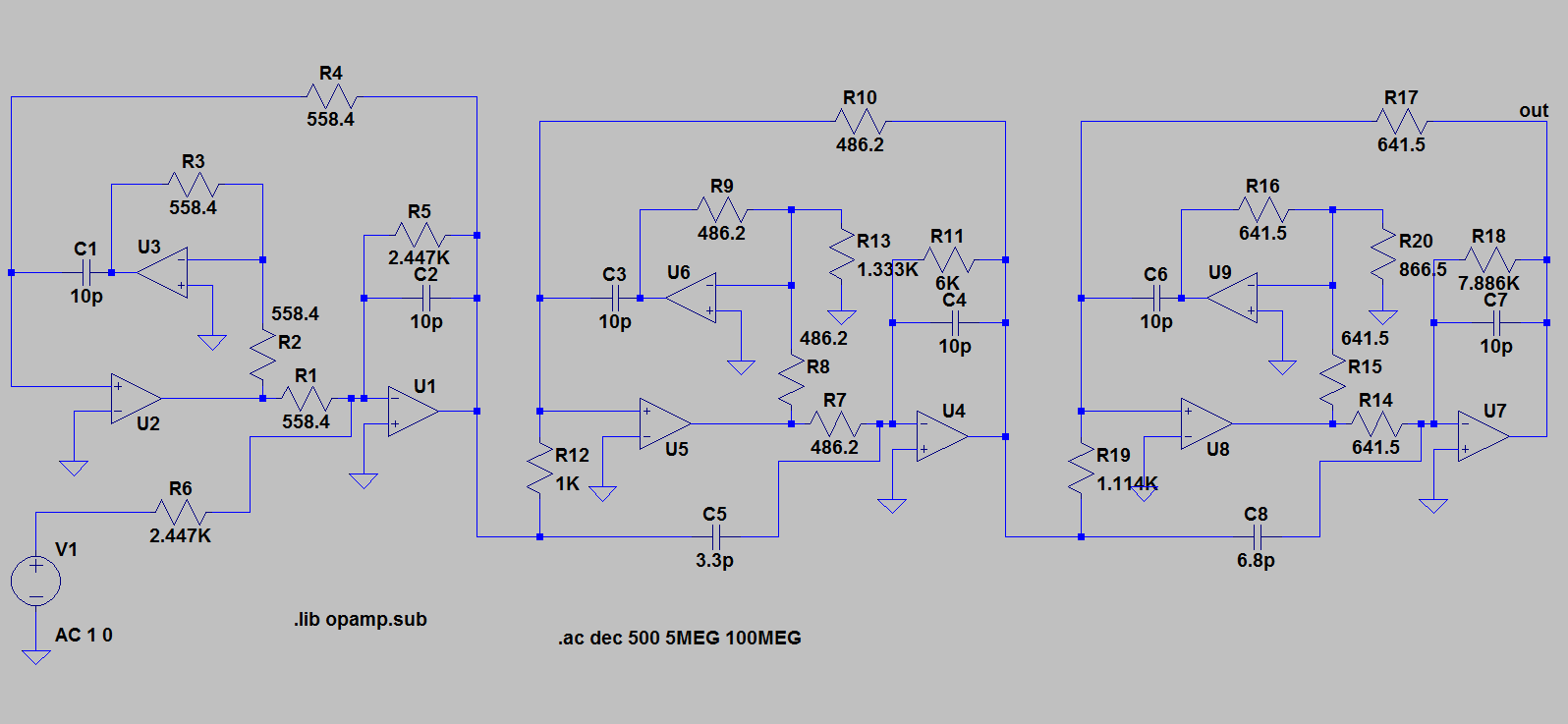Each stage now has 3 operational amplifiers.  We limited the bandwidth of each amplifier to 2 GHz, which is already a very performing amplifier.  The result looks like this: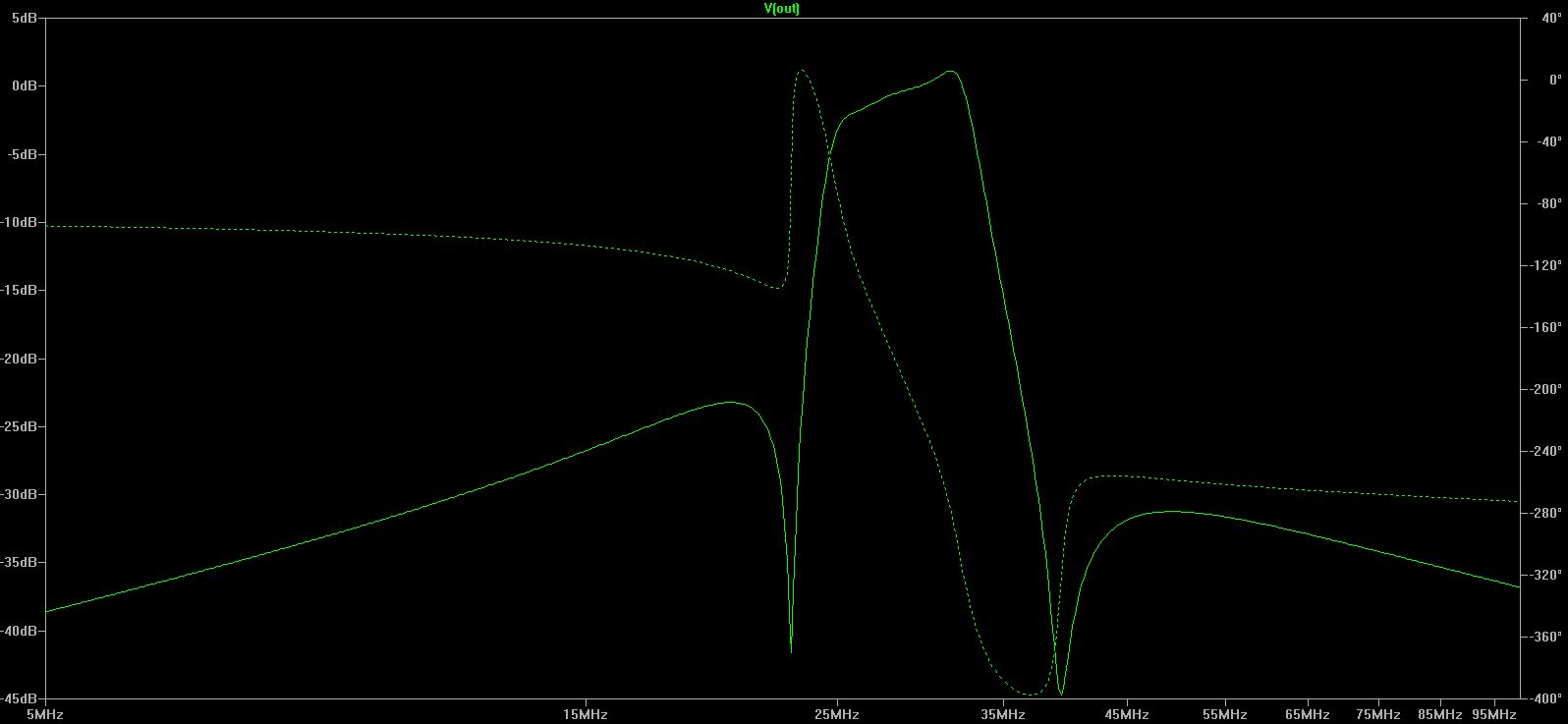As we see, there is a distortion in the pass band.  But at least this filter is realizable, and is not too sensitive to the components.

One really wonders whether one is not better off with a lower-order passive filter than with this complicated active filter with average quality response.

### Digital filters

At first sight on paper, our application would favor an analogue filter.  In as much as a passive filter can be built, it would seem the best solution to our problem: the component values are in very accessible ranges and the filter is not too involved.  The active filter starts to become already a more sophisticated circuit, requiring high-bandwidth quality operational amplifiers and that even a sophisticated circuit gives only half-satisfying results.   In fact, the difficulties for realizing analogue filters reside in the fact that we need a very high frequency selectivity and a flat pass band.  This means that we need high orders which in turn means that we need high precision components.  As we are in the 30 MHz range, in order for the operational amplifiers to have a sufficiently correct behavior, they will need to be high-bandwidth, high gain amplifiers with a GBP of over a few GHz.  Although all this is possible and can be realized, it means that we may consider spending some effort on a digital alternative too.  Of course, as the useful signal is in the 30 MHz range, one would need at least a sample frequency of 100 MHz.   Next it depends on the precision one may need.   Nevertheless, 12 bit, 100 MHz ADC circuits are readily available.   Between a lot of high bandwidth operational amplifiers and some good ADC and processing power, maybe the balance will flip over.

Of course, the signal conditioning at the input will need a more sophisticated anti-aliasing filter than a simple first order filter.  We would propose an active filter, because one has to bring the signal level from the mV level to the dynamics of the digitizer, which is in the Volt range.    Given that we need to let pass signals of the order of 30 MHz, and that we need to cut off signals at 50 MHz, we will need at least a 4th order filter.  But this filter needs to be much less selective than the filters we considered earlier: a 4-th order Butterworth filter will be ok.

In order to treat the data flux of 12 bits every 10 ns (at 100 MHz), I would prefer to use a small FPGA.

However, no matter what digital processing technology is used, the 6-th order Cauer filter that we want to implement, will use the following kind of algorithm.  We will section the filter in a succession of second-order filters.  This has the slight disadvantage of adding a few clock cycles of delay to the availability of the result, but has the big advantage of being numerically much more stable than applying the entire filter equation at once.

A section will take care of two conjugate poles, and two conjugate zeros, and it will take on the following form:

`secin = secin;secin = secin;secin = secinnew;outnew = secin - 2 * zre * secin + (zre * zre + zim * zim) * secin;outnew = outnew + 2 * pre * secout - (pre * pre + pim * pim) * secout;secout = secout;secout = outnew;`

This section is then repeated (with of course each section its own state variables) 6 times:

the first section with the values:

`zre = 0.9727360 and zim = 0.2319154 ; pre = 0.9246654 and pim = 0.3238547`

the second section with the values:

`zre = 0.9561635 and zim = 0.2928334 ; pre = 0.9421167 and pim = 0.3103005`

the third section with the values:

`zre = 0.9533496 and zim = 0.3018683 ; pre = 0.9496072 and pim = 0.3076116`

the fourth section with the values:

`zre = 0.8597706 and zim = 0.5106804 ; pre = 0.9101001 and pim = 0.3570613`

the fifth section becomes:

`zre = 0.9111290 and zim = 0.4121213 ; pre = 0.9130628 and pim = 0.3826558`

and finally the sixth section:

`zre = 0.9163805 and zim = 0.4003083 ; pre = 0.9176718 and pim = 0.3914969`

The result has to be multiplied by 0.0096172 as a final scale factor. Of course it would be smart to replace the calculations of the constants (such as pre * pre + pim * pim) outside of the sample loop.  We only sketch the algorithm, and don't show an actual implementation which depends on hardware choices.  We have about 24 multiplications and 24 additions to perform per sample, which results, at a sample rate of 100 MHz, in about 5 Gflops / s.  This is very high for a single processor, but is no problem for an FPGA implementation as all operations can be done in parallel.  On an Altera family of FPGA, this would take a few 1000 LE, which means that, if it is the only function the FPGA has to perform, we are in the ballpark of 20 - 30 Euro components.

This algorithm gives the same quality of results as the plots of the theoretical cauer filter.   As we see, the digital solution requires some modest investment, but at least the result is guaranteed and of high quality.  Of course we shouldn't forget the anti-aliasing low pass filter which should cut off between 30 MHz and 50 MHz.

### Conclusion

We studied a problem where high frequency selectivity was primordial at moderately high frequencies (tens of MHz).  Traditionally, we are in the range of the discrete passive filter.  Indeed, it is possible to construct relatively selective filters this way, but if we really need high selectivity, the tolerance and non ideality of the components can easily destroy totally the properties of the filter.  In other words, we are very sensitive to the quality of the components, and one shouldn't put one's ambitions too high or the filter will not function with real-world components.

The several 10 MHz domain is less suited to active filters.  The passive components (resistors and small capacitors) aren't the issue any more, but this time the bandwidth of the operational amplifiers is an issue.  If we take a simple topology for the filter, then the requirements for the amplifiers become impossible to satisfy (several tens of GHz GBP).  But even if we take on complex topologies, the results are only of moderate quality even if we take state of the art amplifiers.  In fact, given the effort and quality of components, and the obtained results, one is better off with a less ambitious passive filter.

Finally, we show that the digital solution turns out to be again the most reliable for a reasonable cost if we really need the high selectivity.  But of course, at these frequencies, that cost isn't negligible.

In the end, one should really evaluate how much selectivity is absolutely needed for the application at hand.  The price of overdesigning the selectivity is very large in this case.  If we can go for a moderate selectivity, a passive filter seems adequate.  If we really need high selectivity, then the answer is clearly digital, but at a much higher price and complexity.